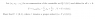# Proving Uncountability of (0,1): A Puzzling Challenge

• aaaa202

## Homework Statement

The problem is attached as a picture.

...

## The Attempt at a Solution

I have been trying a lot to prove this without any really fruitful approach. At first I thought that the statement was false, or that you could at least construct a sequence of rationals such that V=(0,1) the following way:
Let a1 be a real in (0,1). Since the rationals are dense there exists a rational number b1 such that d(a1,b1)<ε. Let this be the first rational number in the sequence. Now let a2 be another real. Because the rationals are dense there exists a rational b2 such that d(a2,b2)<ε/2 etc. etc. and by successive use of this method I could generate the whole (0,1) with my approach. But this fails because (0,1) is not countable. So I'm open for any other approach to this problem.

#### Attachments

•exercise.png
5 KB · Views: 382

## Homework Statement

The problem is attached as a picture.

...

## The Attempt at a Solution

I have been trying a lot to prove this without any really fruitful approach. At first I thought that the statement was false, or that you could at least construct a sequence of rationals such that V=(0,1) the following way:
Let a1 be a real in (0,1). Since the rationals are dense there exists a rational number b1 such that d(a1,b1)<ε. Let this be the first rational number in the sequence. Now let a2 be another real. Because the rationals are dense there exists a rational b2 such that d(a2,b2)<ε/2 etc. etc. and by successive use of this method I could generate the whole (0,1) with my approach. But this fails because (0,1) is not countable. So I'm open for any other approach to this problem.

Can you always construct a sequence from the enumeration that converges to an arbitrary ##x \in (0,1)##?

Also, showing it's a proper subset isn't too bad. Can you think of an element in ##(0,1)## that isn't in ##U##?

The statement that you are trying to prove is false (or, rather, not necessarily true) even after fixing it so that epsilon is fixed and the last line refers to V rather than U.

Edit: The problem, as stated, is very poorly posed. Could you maybe copy it verbatim from the source?